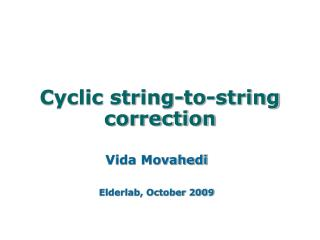DownloadDownload PresentationCyclic string-to-string correction

# Cyclic string-to-string correction

Télécharger la présentation## Cyclic string-to-string correction

- - - - - - - - - - - - - - - - - - - - - - - - - - - E N D - - - - - - - - - - - - - - - - - - - - - - - - - - -
##### Presentation Transcript

1. Cyclic string-to-string correction Vida Movahedi Elderlab, October 2009

2. Contents • Problem Definition • Linear string-to-string correction • Dynamic Programming • Cyclic strings • A faster approach • Application: curve similarity

3. Problem Definition • Two strings: • Edit operation Taking A to B

4. Linear string-to-string correction • Cost of edit • Example: edit ‘high’ to ‘low’ • Edit sequence: delete ‘h’, change ‘i’ to ‘l’, delete ‘g’, change ‘h’ to ‘o’, insert ‘w’ • Goal: find edit sequence with minimum cost

5. Edit Graph, path and trace

6. Dynamic Programming • Why is dynamic programming an option? • Complexity: O(nm)

7. Cyclic strings • Cyclic shifts • Edit cost if cyclic shifts • m possible shifts, m runs of dynamic programming: O(nm2)

8. A faster approach • All edit graphs are included in edit graph of A and BB (let’s call it graph H)

9. Non-crossing Paths • Consider shifts j, k, l where • Traces corresponding to the optimal edit sequences are non-crossing on graph H: P(j), P(k), P(l) • Reducing necessary calculations

10. Non-crossing paths

11. O(nmlogm) algorithm

12. Contour Mapping Distance=7.73 An Application: Curve Similarity • Two curves as two strings A and B • Edit cost: Euclidean distance • Minimum edit cost corresponds to optimal matching • Symmetric cost for each edit operation  Symmetric distance

13. References Maurice Maes (1990), “On a cyclic string-to-string correction problem”, Information Processing Letters, vol. 35, pp. 73-78.